Electron. J. Diff. Equ., Vol. 2016 (2016), No. 151, pp. 1-12.

### Multiple solutions for a fractional p-Laplacian equation with sign-changing potential Vincenzo Ambrosio

Abstract:
We use a variant of the fountain Theorem to prove the existence of infinitely many weak solutions for the fractional p-Laplace equation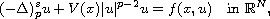where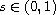,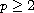,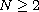,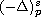is the fractional p-Laplace operator, the nonlinearity f is p-superlinear at infinity and the potential V(x) is allowed to be sign-changing.

Submitted May 10, 2016. Published June 20, 2016.
Math Subject Classifications: 35A15, 35R11, 35J92.
Key Words: Fractional p-Laplacian; sign-changing potential; fountain theorem.

Show me the PDF file (258 KB), TEX file for this article.Vincenzo Ambrosio Dipartimento di Matematica e Applicazioni "R. Caccioppoli" Università degli Studi di Napoli Federico II via Cinthia, 80126 Napoli, Italy email: vincenzo.ambrosio2@unina.it